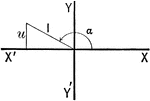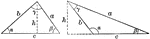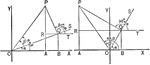### Sum of 2 Acute Angles

Illustration of two triangles, showing the sine of the sum of two acute angles expressed in terms of…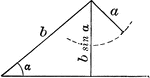### Ambiguous Case

Illustration of one possible outcome (no triangle occurs) when discussing the ambiguous case using the…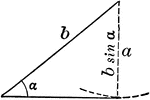### Ambiguous Case

Illustration of one possible outcome (1 triangle occurs) when discussing the ambiguous case using the…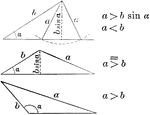### Ambiguous Case

Illustration of one possible outcome (2 triangles occur) when discussing the ambiguous case using the…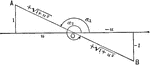### Negative Cotangent Angles

Illustration that can be used to show that if the cotangent of an angle is negative the angle must terminate…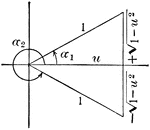### Angle Expressed As An Inverse Function

Illustration that can be used to show that when given an angle, expressed as an inverse function of…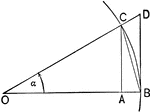### Triangles and Sectors in Quadrant I

Illustration of an angle &alpha with the vertex at the center, O, of a circle with radius OB. AC and…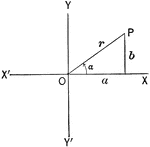Illustration of an angle &alpha with the terminal side used to draw a triangle in quadrant I.